# Tips from the blog III – violin plots

Having recently got my head around violin plots, I thought I would explain what they are and why you might want to use them.

There are several options when it comes to plotting summary data. I list them here in order of granularity, before describing violin plots and how to plot them in some detail.

Bar chart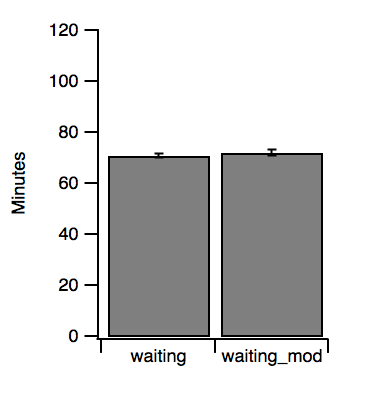This is the mainstay of most papers in my field. Typically, a bar representing the mean value that’s been measured is shown with an error bar which shows either the standard error of the mean, the standard deviation, or more rarely a confidence interval. The two data series plotted in all cases is the waiting time for Old Faithful eruptions (waiting), a classic dataset from R. I have added some noise to waiting (waiting_mod) for comparison. I think it’s fair to say that most people feel that the bar chart has probably had its day and that we should be presenting our data in more informative ways*.

Pros: compact, easy to tell differences between groups

Cons: hides the underlying distribution, obscures the n number

Box plot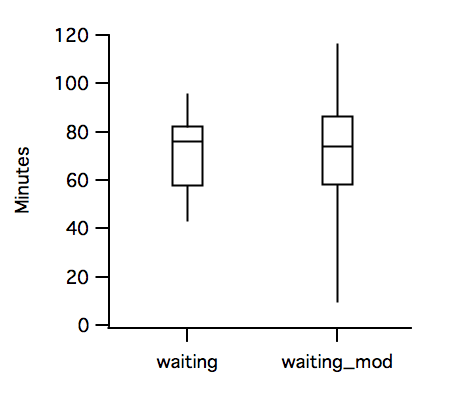The box plot – like many things in statistics – was introduced by Tukey. It’s sometimes known as a Tukey plot, or a box-and-whiskers plot. The idea was to give an impression of the underlying distribution without showing a histogram (see below). Histograms are great, but when you need to compare many distributions they do not overlay well and take up a lot of space to show them side-by-side. In the simplest form, the “box” is the interquartile range (IQR, 25th and 75th percentiles) with a line to show the median. The whiskers show the 10th and 90th percentiles. There are many variations on this theme: outliers can be shown or not, the whiskers may show the limits of the dataset (or something else), the boxes can be notched or their width may represent the sample size…

Pros: compact, easy to tell differences between groups, shows normality/skewness

Cons: hides multimodal data, sometimes obscures the n number, many variations

Histogram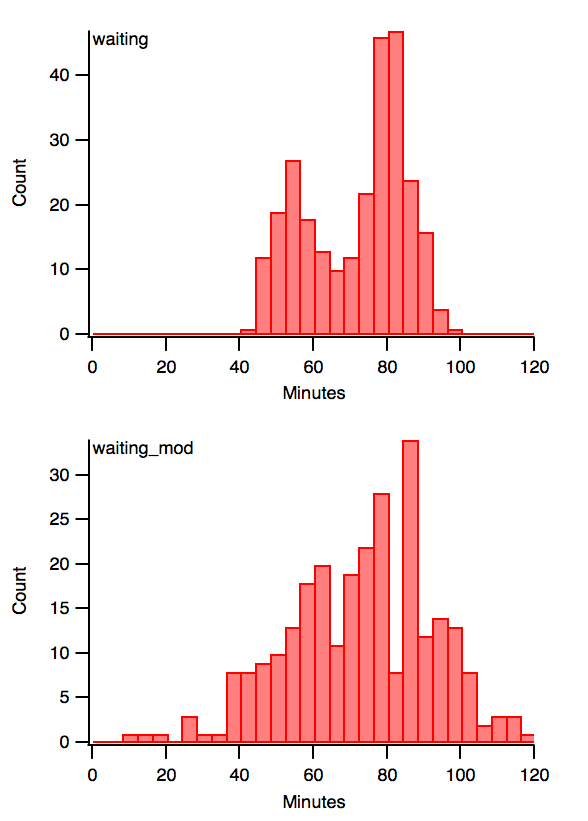A histogram is a method of showing the distribution of a dataset and was introduced by Pearson. The number of observations within a bin are counted and plotted. The ‘bars’ sit next to each other, because the variable being measured is continuous. The variable being measured is on the x-axis, rather than the category (as in the other plots).

Often the area of all the bars is normalised to 1 in order to assess the distributions without being confused by differences in sample size. As you can see here, “waiting” is bimodal. This was hidden in the bar chart and in the bot plot.

Related to histograms are other display types such as stemplots or stem-and-leaf plots.

Pros: shows multimodal data, shows normality/skewness clearly

Cons: not compact, difficult to overlay, bin size and position can be misleading

Scatter dot plotIt’s often said that if there are less than 10 data points, then best practice is to simply show the points. Typically the plot is shown together with a bar to show the mean (or median) and maybe with error bars showing s.e.m., s.d., IQR. There are a couple of methods of plotting the points, because they need to be scattered in x value in order to be visualised. Adding random noise is one approach, but this looks a bit messy (top). A symmetrical scatter can be introduced by binning (middle) and a further iteration is to bin the y values rather than showing their true location (bottom). There’s a further iteration which constrains the category width and overlays multiple points, but again the density becomes difficult to see.

These plots still look quite fussy, the binned version is the clearest but then we are losing the exact locations of the points, which seems counterintuitive. Another alternative to scattering the dots is to show a rug plot (see below) where there is no scatter.

Pros: shows all the observations

Cons: can be difficult to assess the distribution

Violin plotThis type of plot was introduced in the software package NCSS in 1997 and described in this paper: Hintze & Nelson (1998) The American Statistician 52(2):181-4 [PDF]. As the title says, violin plots are a synergism between box plot and density trace. A thin box plot is shown together with a symmetrical kernel density estimate (KDE, see explanation below). The point is to be able to quickly assess the distribution. You can see that the bimodality of waiting in the plot, but there’s no complication of lots of points just a smooth curve to see the data.

Pros: shows multimodal data, shows normality/skewness unambiguously

Cons: hides n, not familiar to many readers.

* Why is the bar chart so bad and why should I show my data another way?The best demonstration of why the bar chart is bad is Anscombe’s Quartet (the figure to the right is taken from the Wikipedia page). These four datasets are completely different, yet they all have the same summary statistics. The point is, you would never know unless you plotted the data. A bar chart would look identical for all four datasets.

Making Violin Plots in IgorPro

I wanted to make Violin Plots in IgorPro, since we use Igor for absolutely everything in the lab. I wrote some code to do this and I might make some improvements to it in the future – if I find the time! This was an interesting exercise, because it meant forcing myself to understand how smoothing is done. What follows below is an aide memoire, but you may find it useful.

What is a kernel density estimate?

A KDE is a non-parametric method to estimate a probability density function of a variable. A histogram can be thought of as a simplistic non-parametric density estimate. Here, a rectangle is used to represent each observation and it gets bigger the more observations are made.

What’s wrong with using a histogram as a KDE?The following examples are taken from here (which in turn are taken from the book by Bowman and Azzalini described below). A histogram is simplistic. We lose the location of each datapoint because of binning. Histograms are not smooth and the estimate is very sensitive to the size of the bins and also the starting location of the first bin. The histograms to the right show the same data points (in the rug plot).Using the same bin size, they result in very different distributions depending on where the first bin starts. My first instinct to generate a KDE was to simply smooth a histogram, but this is actually quite inaccurate as it comes from a lossy source. Instead we need to generate a real KDE.

How do I make a KDE?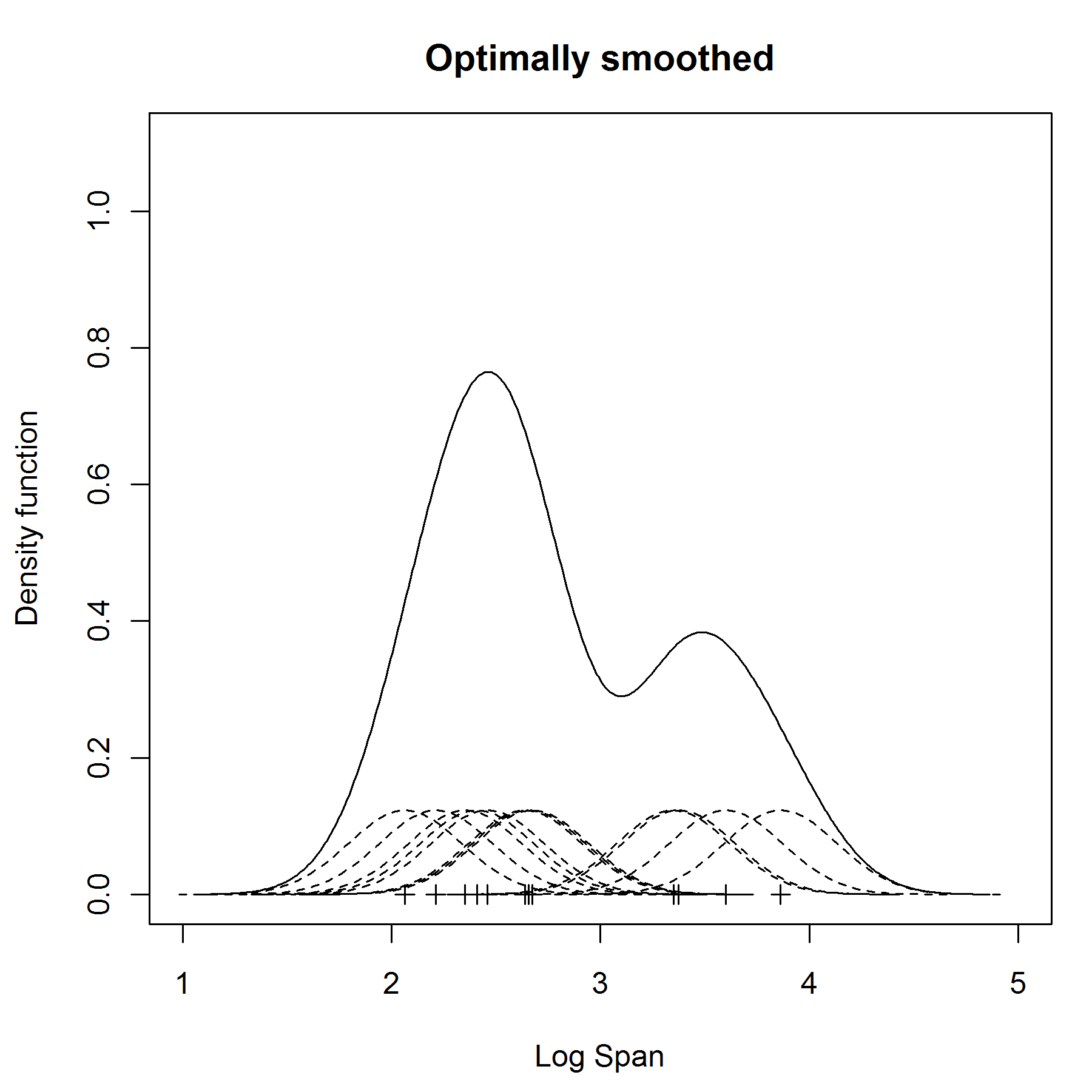To do this we place a kernel (a Gaussian is commonly used) at each data point. The rationale behind this is that each observation can be thought of as being representative of a greater number of observations. It sounds a bit bizarre to assume normality to estimate a density non-parametrically, but it works. We can sum all of the kernels to give a smoothed distribution: the KDE. Easy? Well, yes as long as you know how wide to make the kernels. To do this we need to find the bandwidth, h (also called the smoothing parameter).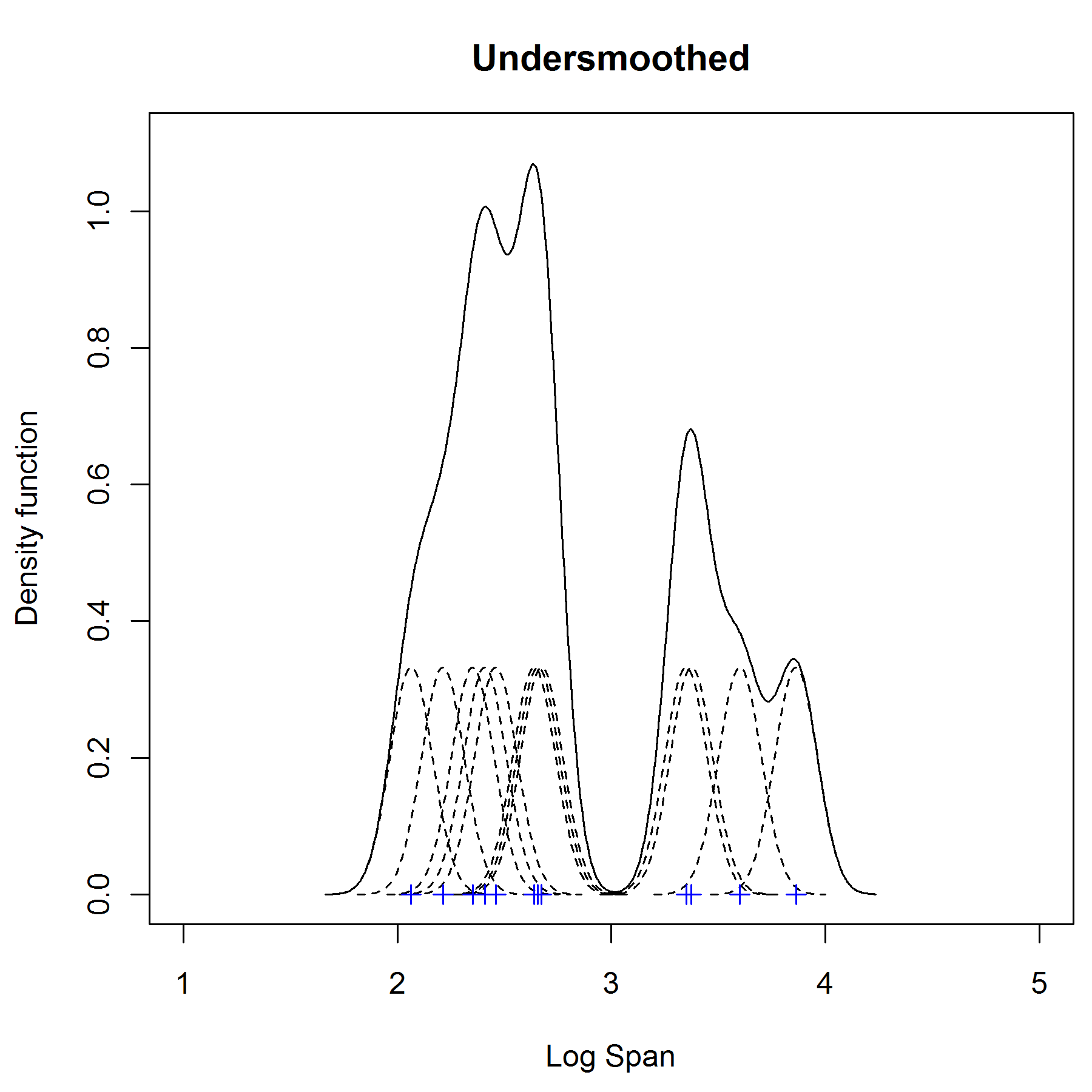It turns out that this is not completely straightforward. The answer is summarised in this book: Bowman & Azzalini (1997) Applied Smoothing Techniques for Data Analysis. In the original paper on violin plots, they actually do not have a good solution for selecting h for drawing the violins, and they suggest trying several different values for h. They recommend starting at ~15% of the data range as a good starting point. Obviously if you are writing some code, the process of selecting h needs to be automatic.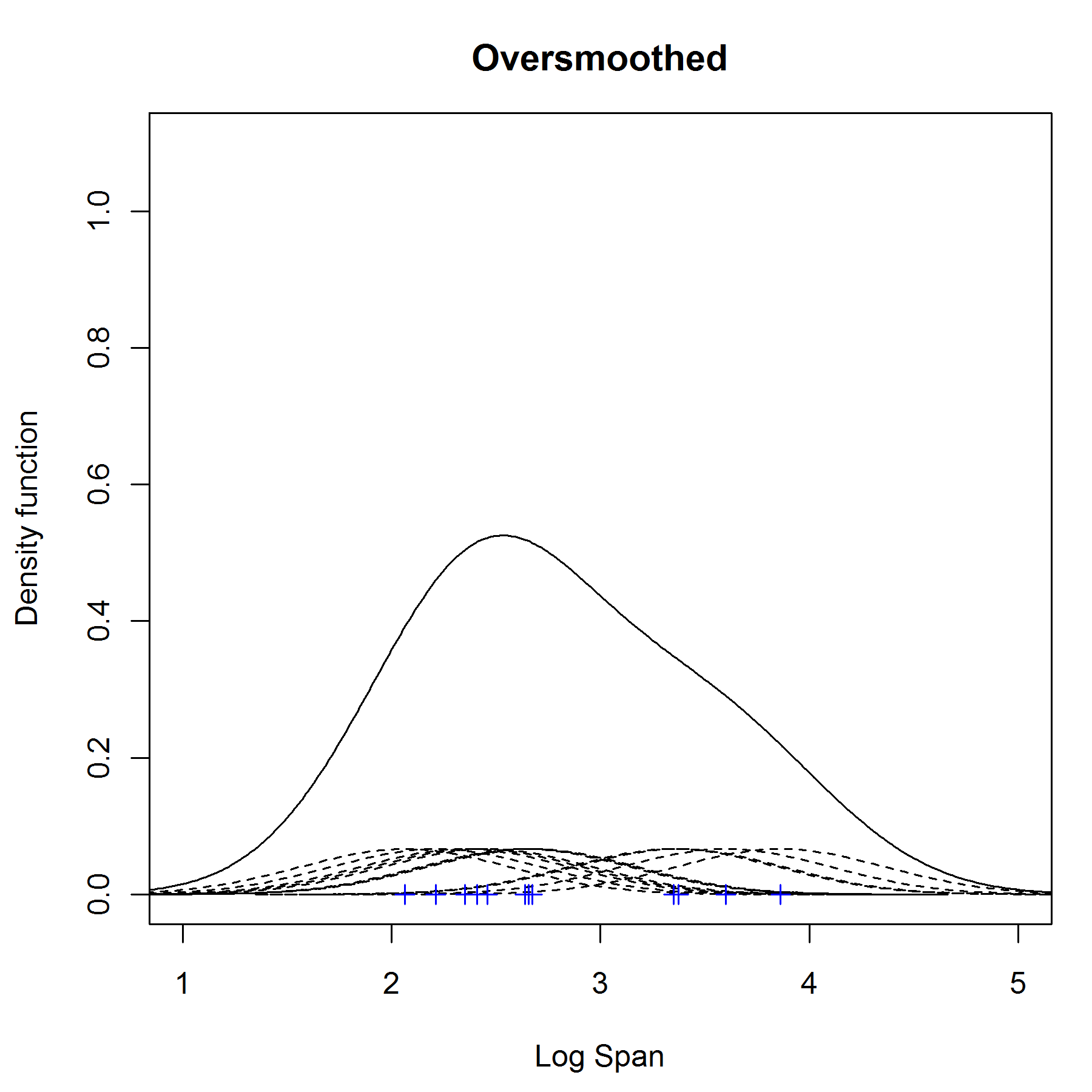Optimising h is necessary because if h is too large, the estimate with be oversmoothed and features will be lost. If is too small, then it will be undersmoothed and bumpy. The examples to the right (again, taken from Bowman & Azzalini, via this page) show examples of undersmoothed, oversmoothed and optimal smoothing.

An optimal solution to find h is

$$h = \left(\frac{4}{3n}\right)^{\frac{1}{5}}\sigma$$

This is termed Silverman’s rule-of-thumb. If smoothing is needed in more than one dimension, the multidimensional version is

$$h = \left\{\frac{4}{\left(p+2\right)n}\right\}^{\frac{1}{\left(p+4\right)}}\sigma$$You might need multidimensional smoothing to contextualise more than one parameter being measured. The waiting data used above describes the time to wait until the next eruption from Old Faithful. The duration of the eruption is measured, and also the wait to the next eruption can be extracted, giving three parameters. These can give a 3D density estimate as shown here in the example.

The Bowman & Azzalini recommend that, if the distribution is long-tailed, using the median absolute deviation estimator is robust for $$\sigma$$.

$$\tilde\sigma=median\left\{|y_i-\tilde\mu|\right\}/0.6745$$

where $$\tilde\mu$$ is the median of the sample. All of this is something you don’t need to worry about if you use R to plot violins, the implementation in there is rock solid having been written in S plus and then ported to R years ago. You can even pick how the h selection is done from sm.density, or even modify the optimal h directly using hmult.

To get this working in IgorPro, I used some code for 1D KDE that was already on IgorExchange. It needed a bit of modification because it used FastGaussTransform to sum the kernels as a shortcut. It’s a very fast method, but initially gave an estimate that seemed to be undersmoothed. I spent a while altering the formula for h, hence the detail above. To cut a long story short, FastGaussTransform uses Taylor expansion of the Gauss transform and it just needed more terms to do this accurately. This is set with the /TET flag. Note also, that in Igor the width of a Gauss is sigma*2^1/2.

OK, so how do I make a Violin for plotting?

I used the draw tools to do this and placed the violins behind an existing box plot. This is necessary to be able to colour the violins (apparently transparency is coming to Igor in IP7). The other half of the violin needs to be calculated and then joined by the DrawPoly command. If the violins are trimmed, i.e. cut at the limits of the dataset, then this required an extra point to be added. Without trimming, this step is not required. The only other issue is how wide the violins are plotted. In R, the violins are all normalised so that information about n is lost. In the current implementation, box width is 0.1 and the violins are normalised to the area under the curve*(0.1/2). So, again information on n is lost.

Future improvements

Ideas for developments of the Violin Plot method in IgorPro

• incorporate it into the ipf for making boxplots so that it is integrated as an option to ‘calculate percentiles’
• find a better solution for setting the width of the violin
• add other bandwidth options, as in R
• add more options for colouring the violins

What do you think? Did I miss something? Let me know in the comments.

References

Bowman, A.W. & Azzalini, A. (1997) Applied Smoothing Techniques for Data Analysis : The Kernel Approach with S-Plus Illustrations: The Kernel Approach with S-Plus Illustrations. Oxford University Press.

Hintze, J.L. & Nelson, R.D. (1998) Violin plots: A Box Plot-Density Trace Synergism. The American Statistician, 52:181-4.